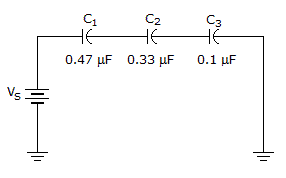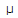# Electronics - Capacitors - Discussion

### Discussion :: Capacitors - General Questions (Q.No.22)

22.What is the capacitance of the circuit shown in the given circuit?

 [A]. 0.066F [B]. 0.9F [C]. 65.97 pF [D]. 900 pF

Explanation:

No answer description available for this question.

 Ravi V said: (May 22, 2011) C1+C2+C3 = 0.47+0.33+0.1 = 0.9

 Anas said: (Jun 8, 2011) 1/c1+1/c2+1/c3=.066

 Faiz Ullah said: (Aug 11, 2011) 1/(1/C1+1/C2+1/C3)=0.06600

 Pawan Jangra said: (Sep 12, 2011) When Two or more than Two capacitors are in series then Total capacitance is :- T.c = 1/c1+1/c2+1/c3.... = 1/0.47+1/0.33+1/0.1 = 0.066

 Dwa said: (Jul 22, 2012) @ravi ur answer is rite for parallel connection.

 Nagesh said: (Sep 9, 2012) @Dwa your explanation is correct.

 Vasanthi.M said: (Sep 17, 2012) If circuit shows the capacitors connetced in series so formula is ct = (c1*c2)+(c2*c3)+(c3*c1)/c1*c2*c3 = (0.47*0.33)+(0.33*0.1)+(0.1*0.47)/0.47*0.33*0.1 = 0.1551+0.33+0.47/0.1551 = 0.2351/0.1551 = 0.659micro farad

 Gomathi said: (Jan 16, 2014) The formula is reverse to the resistor so, 1/c = (1/c1+1/c2+1/c3). = (1/0.47)+(1/0.33)+(1/0.1). = 15.15. c = 1/15.15. c = 0.06microfarad.

 Vijayakumar said: (Jul 5, 2014) Here capacitor is in series so c1+c2+c3.. So ans is 0.9.....1/c1+1/c2+1/c3 is parallel formula.

 Armandwish said: (Sep 23, 2015) You can do it in resistance parallel formula also.

 Karthi said: (Oct 19, 2016) @Ravi is wrong. And @Anas is right.